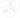## 最团结的圆

Sat, 1st February 2020Edit on Github三角形极值

# 问题来源

2019年1月lsr314提问，如图: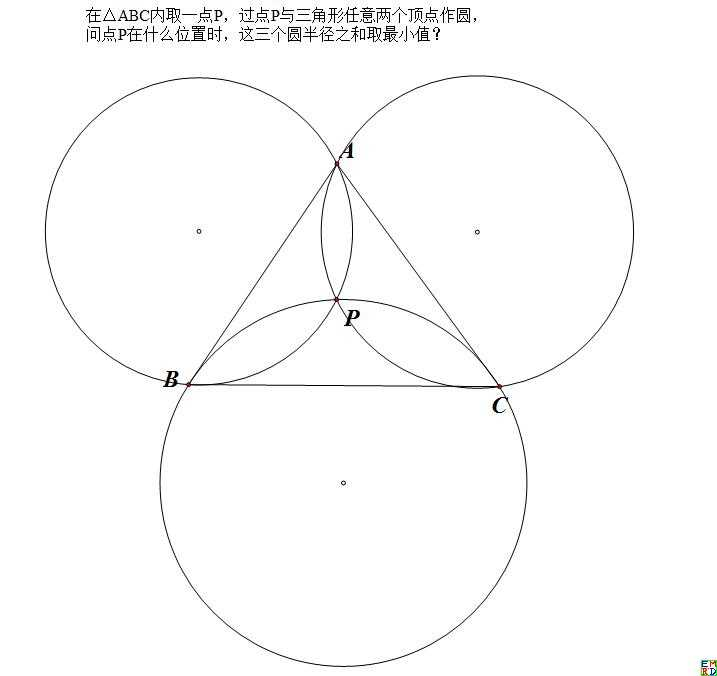# 极值点分析

lsr314首先给出了一个结论: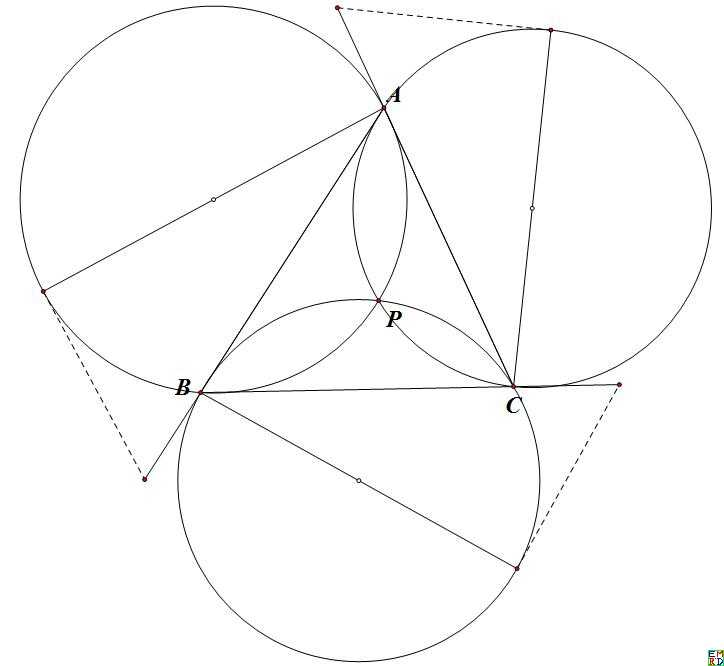mathe验算并赞同lsr314的结论：

$u=\cos(x),v=\cos(y),w=\cos(z)$,

$\begin{cases}u^2+v^2+w^2-1-2uvw=0\\ 1-u^2-ahu=0\\ 1-v^2-bhv=0\\ 1-w^2-chw=0 \end{cases}$

$h$满足的方程为$6534375h^7 - 2098350h^6 + 2379375h^5 + 70000h^4 + 66360h^3 + 137600h^2 - 18000h + 9856=0$.
mathe并且使用在线magma计算上面方程的Galios群得出
Symmetric group G acting on a set of cardinality 7
Order = 5040 = $2^4\times 3^2 \times 5 \times 7$

lsr314进一步提问除了mathe前面提及三角形内部的极值点，是否还存在外部极值点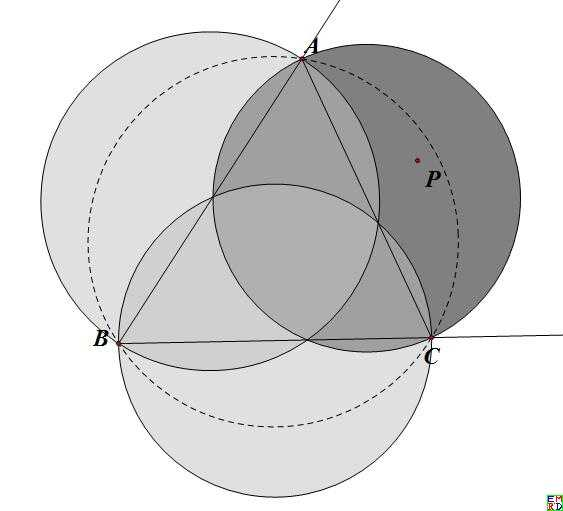mathe也进一步分析这种情况，发现外部极值点和内部极值点关系密切:

$u=v=-0.55594708911635773652783798763298602680,w=-0.38184566820609717835179976754753014669$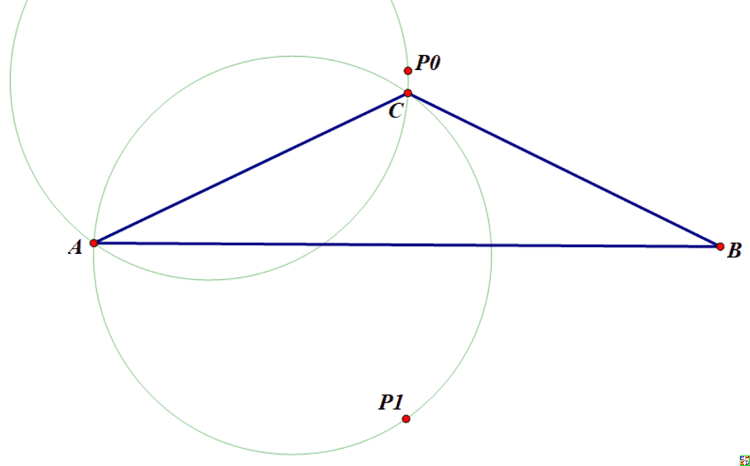mathe还尝试研究$P_0$从三角形内部变换到外部的边界条加你，其结果很复杂,是一个关于a,b,c的16次方程。 而如果设置$a=b$,那么边界条件化为：
$c^{16} - 14a^2c^{14} + 91a^4c^{12} - 348a^6c^{10} + 842a^8c^8 - 1296a^{10}c^6 + 1184a^{12}c^4 - 512a^{14}c^2=0$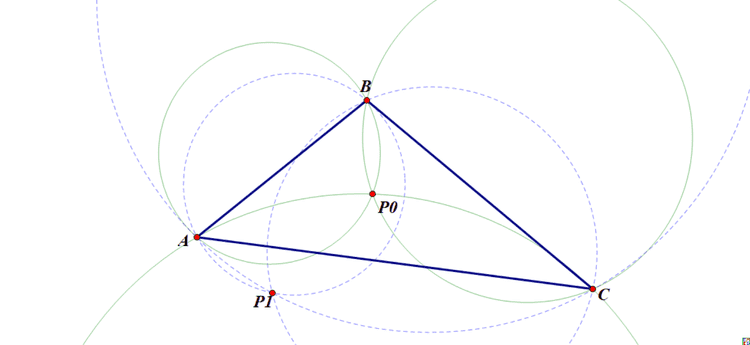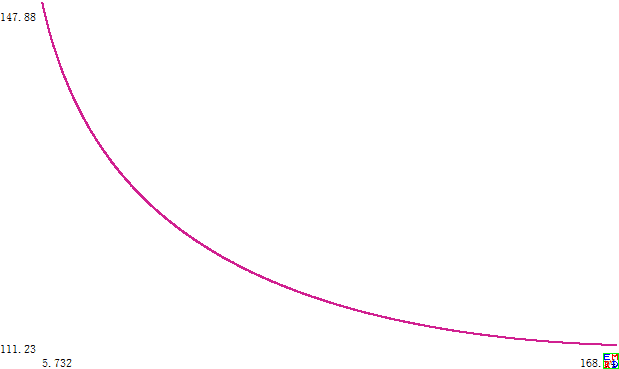dlpg070认为极值点应该还是四个而不是两个: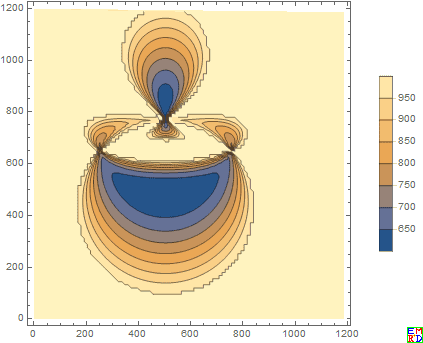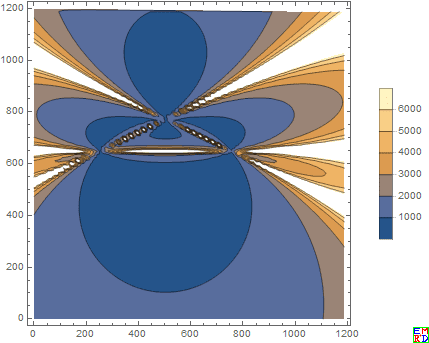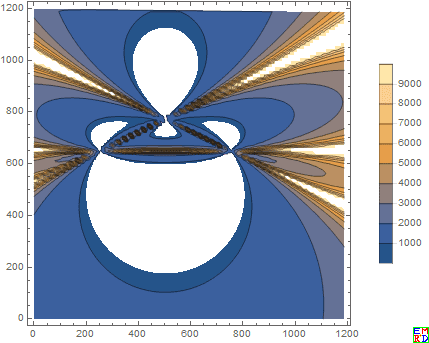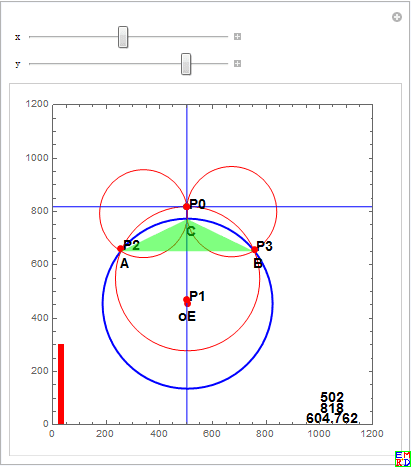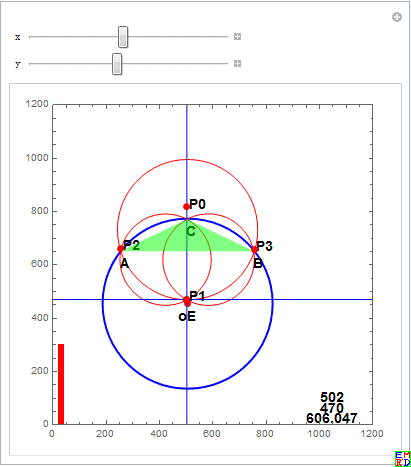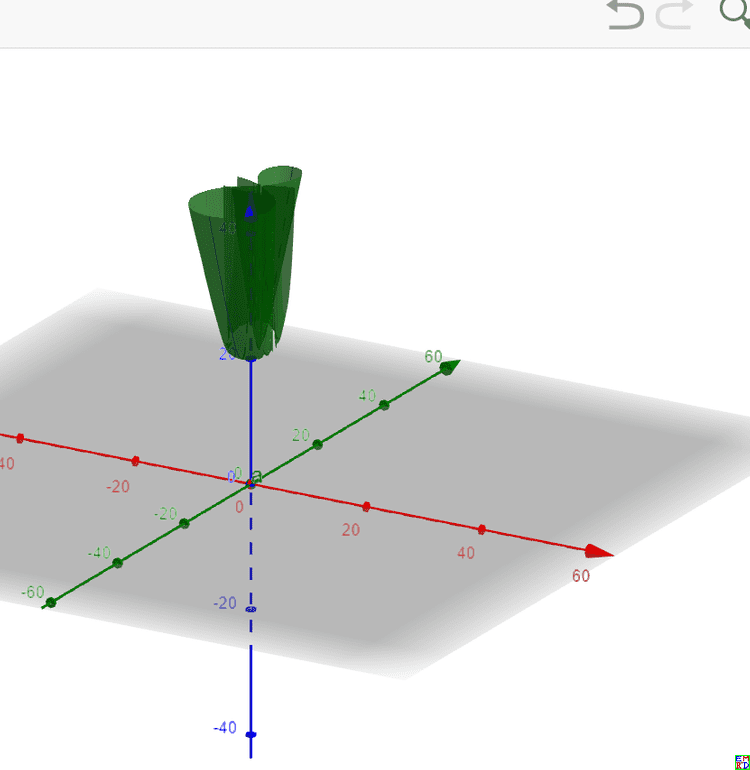# 派生几何变换

mathe发现给定平面上一个三角形ABC,对于平面上任意一个点P(P不在三角形边上），分别做三角形P关于BC,CA,AB的对称点$P_1,P_2,P_3$,那么三角形$BCP_1,CAP_2,ABP_3$的外接圆交于一点Q,这种方式确定了一个$P\to Q$的一一对映关系，请问这是一种什么样的对应关系？ 用几何画板做了个特殊情况的$P\to Q$轨迹，结果证明不是线性的对合变换，因为直线被变换为奇怪的曲线了：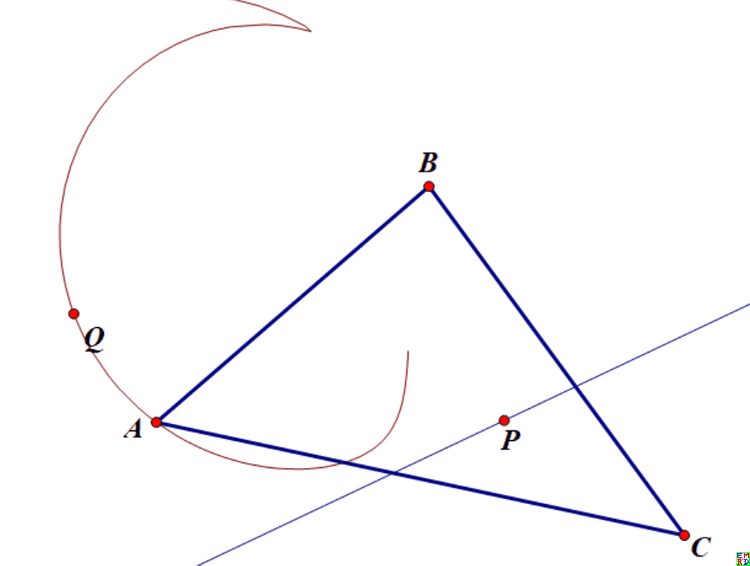lsr314发现原三角形外接圆，$P_0$关于三边对称点外接圆和$P_1$关于三边对称点外接圆三圆共弦：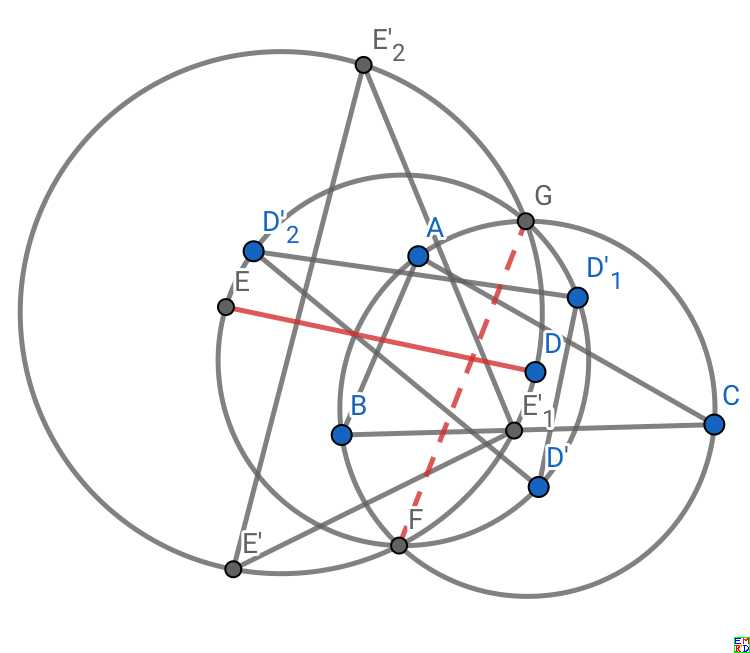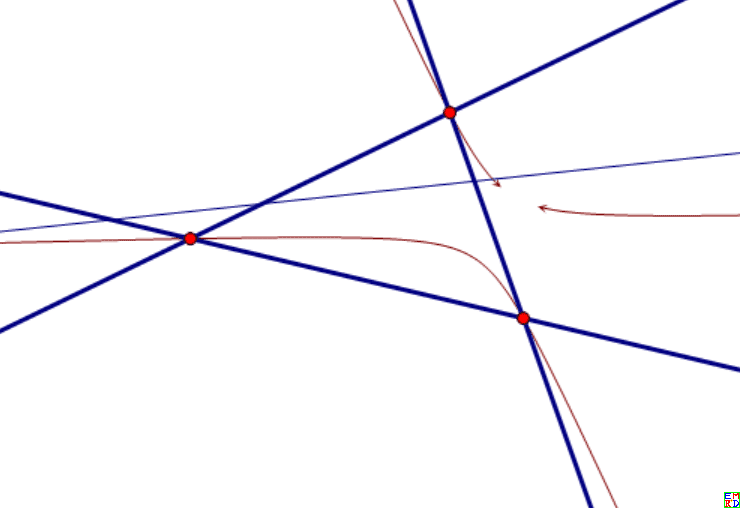hujunhua指出这个变换边上的点被映射到相对的顶点，故由映射的连续性可知三角形内的点是映射到三角形内的。

hujunhua发现一种有意思的几何变换存在周期迭代:

mathe试着把三角形推广到四边形结果发现还是存在周期现象，只是现在是以4为周期：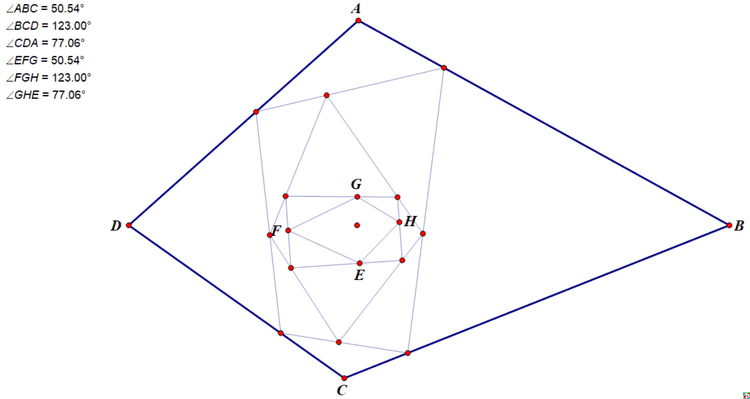mathe继续提供Pari/Gp代码计算更多边的情况，证实也都存在周期现象。

(11:12) gp > m=granm(10,2);
(11:12) gp > h=m;
(11:12) gp > for(u=1,10,h=perprotate(h))
(11:12) gp > pangle(m)
%58 = [0.13094390757425572586897330693922760638, 0.27478457799382083099586956163919994750, -0.83403509336655614549647414732045555854, 0.59735297479132672647706922853304549911, -0.52632380321904226169862901627243144170, -0.80511734034786098563793583703215670988, -0.38438818934646934888818443410206486840, -0.99130533701336277684115213150606694557, 0.41861095048399809858695131599839686302, -0.066203186117102511991044546302214391115]
(11:13) gp > pangle(h)
%59 = [0.13094390757425572586897330693922760656, 0.27478457799382083099586956163919994285, -0.83403509336655614549647414732045555834, 0.59735297479132672647706922853304549645, -0.52632380321904226169862901627243144215, -0.80511734034786098563793583703215671111, -0.38438818934646934888818443410206487575, -0.99130533701336277684115213150606694557, 0.41861095048399809858695131599839686347, -0.066203186117102511991044546302214393602]

P在多边形内部时，P和各顶点连线把初始多边形的相应内角分为两部分，这2n个角度集合记为S。

n个符号的简单循环，周期自然是n.
P于多边形各边的镜像点可以由P点在各边上的投影来代替，这只不过将诸镜像点构成的多边形以P为位似中心缩小了一半。 引理：如图，P为角ABC内一点，E、F为P在两边上的投影（ $\theta=\frac{\pi}2$)，显然B、E、P、F四点共圆，故有图中标示的两组等角B1和B2（角B的两部分）。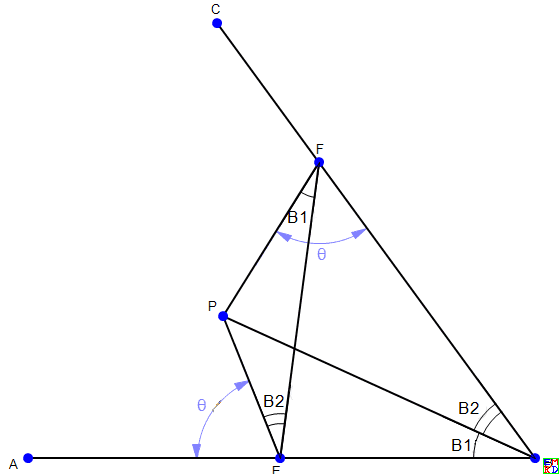$S_1=\{V_{i,1}\}, S_2=\{V_{i,2}\},S=S_1\cup S_2$.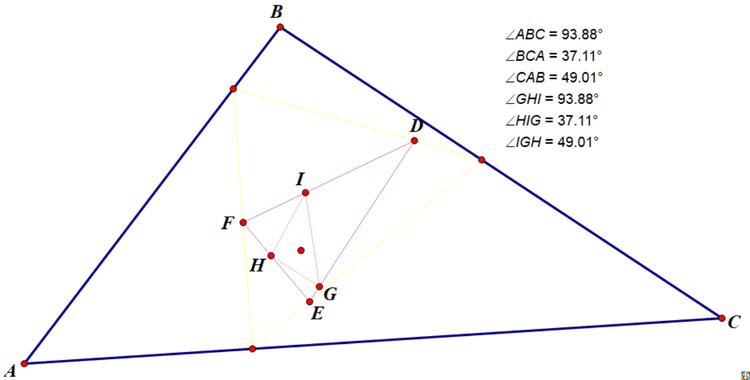hujunhua尝试另外一种扩展

lsr314指出，作图验证这时对椭圆就不成立了：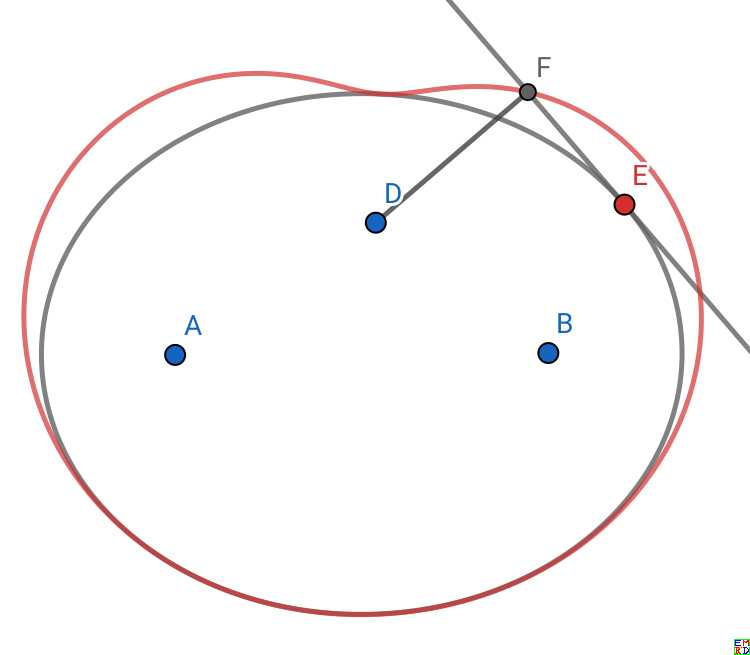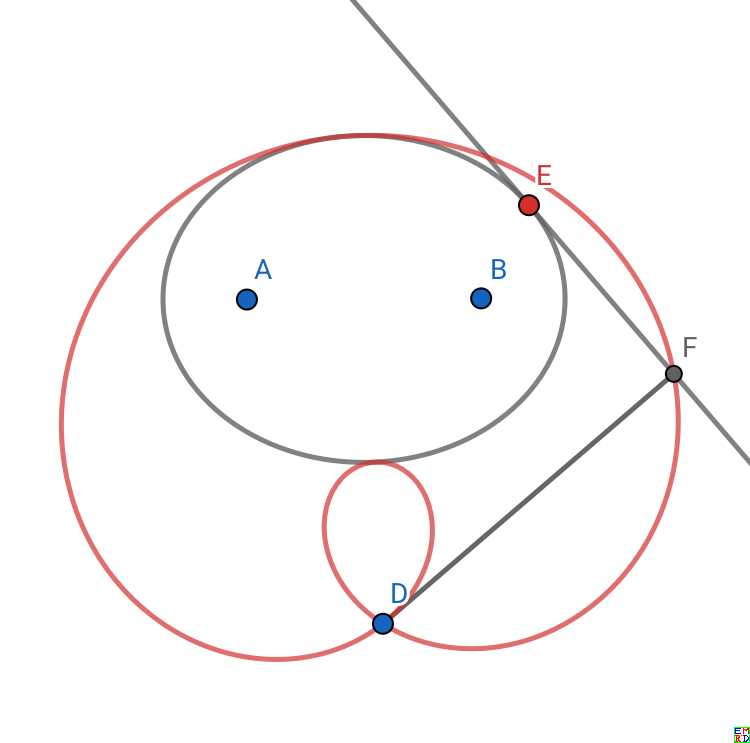wayne也来凑热闹，给出了另外一种漂亮的图片: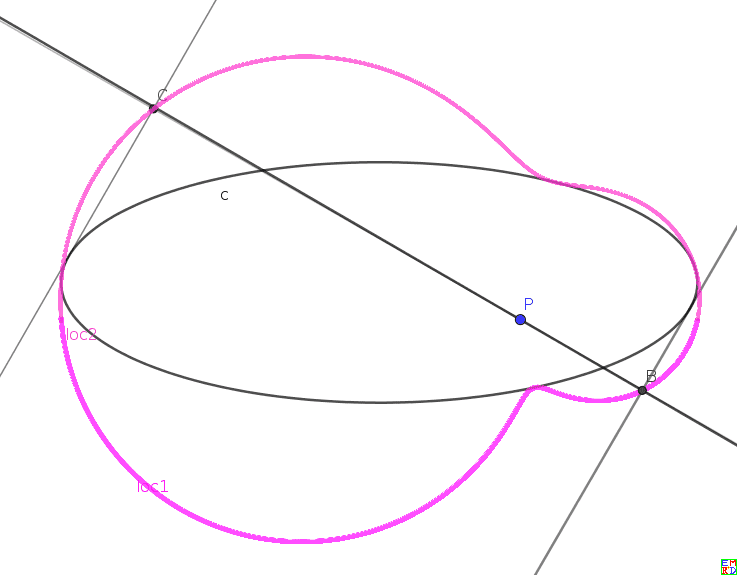Github